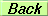# SEMINAR

Reparametrization Invariance, the controversial extraction of $alpha$ from $B opipi$ and New Physics

Dr. Miguel Nebot

(CFTP-IST)

Sala P8, IST, Edifício Matemática
Thursday, May 24th, 2007 at 03:00 PM

Abstract

The extraction of the weak phase $\alpha$ from $B\to\pi\pi$ decays has been controversial from a statistical point of view, as illustrated by the frequentist vs. bayesian confrontation. Analyses of several relevant questions which were not deserving full attention and pervade the extraction of $\alpha$ are presented. Reparametrization Invariance proves appropriate to understand those issues. It is shown how some Standard Model inspired parametrizations can be senseless or inadequate if they go beyond the minimal Gronau & London assumptions: the single weak phase $\alpha$ just in the $\Delta I=3/2$ amplitudes, the isospin relations and experimental data. Beside those analyses, we extract $\alpha$ through the use of several adequate parametrizations, showing that there is no relevant discrepancy between frequentist and bayesian results. The most relevant information, in terms of $\alpha$, is the exclusion of values around $\alpha\sim\pi/4$; this result is valid in the presence of arbitrary New Physics contributions to the $\Delta I=1/2$ piece.